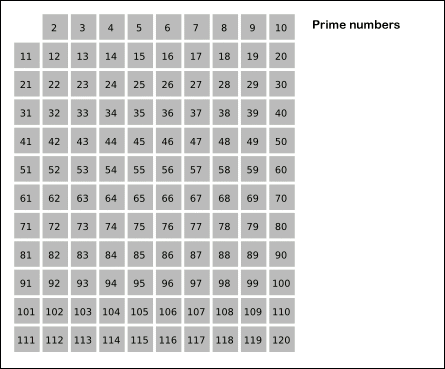Sieve of Eratosthenes is an algorithm for making tables of primes. In this algorithm we sequentially write down the integers from 2 to the highest number n we wish to include in the table. Cross out all numbers >2 which are divisible by 2 (every second number). Find the smallest remaining number >2. It is 3. So cross out all numbers >3 which are divisible by 3 (every third number). Find the smallest remaining number >3. It is 5. So cross out all numbers >5 which are divisible by 5 (every fifth number) and so on. At the end of this algorithm, all unmarked numbers are prime numbers.Now, I observed that only first 4 prime no. are needed to find all prime no less than 100 (120 to be exact). This makes me wonder, if I choose only first N prime no. for my sieve generation then what will be the upper limit of my table, i.e. first composite no which will be identified as prime no if we use only first N prime no. for sieve generation.

Input Specifications
First line will contain no. of test cases T.
T lines follow. Each line will contain only one integer N

Output Specifications
Output first composite no which will be identified as prime no, if we use only first N prime no. for sieve generation, for each test case on separate lines.

Constrains
``` T <= 70001 N <= 70000 (First 70000 prime no are < 10^6) ```

Sample Input
``` 1 4 ```

Sample Output
``` 121 ```

Problem Setter : Pushkar Anand

Languages: Brain,C,C++,Java,C#,JavaScript,Pascal,Perl,PHP,Python,Ruby,Text

Time Limit: 2 Second(s)
Score: 100 Point(s)
Input File Limit: 50000 Bytes

Mode Judge

RankNameScore
1xyz0
2Ams0
3TIP0
4team420
5xyzz0
6asdasdasd0
7abcd0
8khankhan0
9Gabriel0
10gigel0